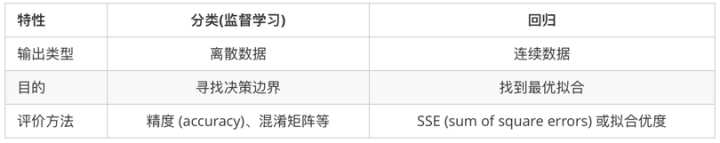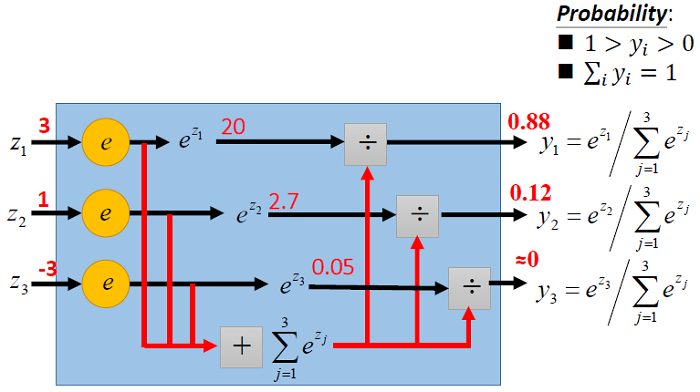• 输入变量与输出变量均为连续变量的预测问题是回归问题
• 输出变量为有限个离散变量的预测问题为分类问题
• 输入变量与输出变量均为变量序列的预测问题为标注问题

• 预测明天的气温是多少度，这是一个回归任务；
• 预测明天是阴、晴还是雨，就是一个分类任务。## Logistic Regression

Logistic Regression与softmax回归的区别如下：

• logistic回归（是非问题）中，$y^i$取0或者1；
• softmax回归（多分类问题）中，$y^i$取$1,2…k$中的一个表示类别标号的一个数（假设共有$k$类）。

### 交叉熵损失函数

• $n$为类别的数量
• $y_j$为指示变量（0或1）,如果该类别$j$和观测样本的类别相同就是1，否则是0；
• $a_j$对于观测样本属于类别$j$的预测概率。

$m$是样本数，$n$是分类个数。其中，$y_{ij}$为指示变量（0或1）,如果样本$i$的类别为$j$就是1，否则是0；$a_{ij}$表示样本$i$属于类别$j$的预测概率。

## softmax回归

Softmax回归处理多分类问题，不同于逻辑回归中$y^{(i)}$表示一个数$\{0,1\}$，这里的$y^{(i)} \in\{1,2, \ldots, k\}$。要搭配one-hot编码使用

softmax回归主要是估算对于输入样本$x^{(i)}$属于每一类别的概率，所以softmax回归的假设函数如下：### logistic、softmax回归和神经网络关系### 参数冗余

Softmax 回归有一个不寻常的特点：它有一个“冗余”的参数集。为了便于阐述这一特点，假设我们从参数向量$\theta_j$减去向量$\psi$这时，每一个$\theta_j$都变成了$\theta_{j}-\psi(j=1,2, \ldots k)$，此时假设函数变成了以下的式子：

## 多项式回归## 参考

------ 本文结束------